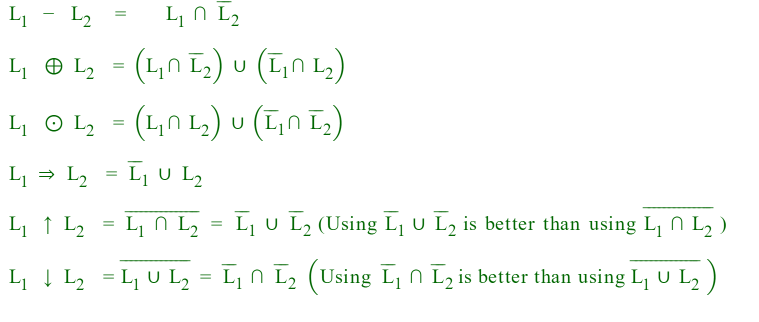# Closure properties Table in TOC

• Difficulty Level : Easy
• Last Updated : 22 Sep, 2022

The Below Table shows the Closure Properties of Formal Languages :

REG = Regular Language
DCFL = deterministic context-free languages,
CFL = context-free languages,
CSL = context-sensitive languages,
RC = Recursive.
RE = Recursive Enumerable

Consider L and M are regular languages :

1. The Kleene star –
∑*, is a unary operator on a set of symbols or strings, ∑, that gives the infinite set of all possible strings of all possible lengths over ∑ including λ.
2. Kleen Plus –
The set ∑+ is the infinite set of all possible strings of all possible lengths over ∑ excluding λ.
3. Complement –
The complement of a language L (with respect to an alphabet E such that E* contains L) is E*–L. Since E* is surely regular, the complement of a regular language is always regular.
4. Reverse Operator –
Given language L, LR is the set of strings whose reversal is in L.
5. Complement –
The complement of a language L (with respect to an alphabet E such that E* contains L) is E*–L. Since E* is surely regular, the complement of a regular language is always regular.
6. Union –
Let L and M be the languages of regular expressions R and S, respectively. Then R+S is a regular expression whose language is(L U M).
7. Intersection –
Let L and M be the languages of regular expressions R and S, respectively, then it is a regular expression whose language is L intersection M.
8. Set Difference operator –
If L and M are regular languages, then so is L – M = strings in L but not M.
9. Homomorphism –
A homomorphism on an alphabet is a function that gives a string for each symbol in that alphabet.
10. Inverse Homomorphism –
Let h be a homomorphism and L a language whose alphabet is the output language of h. h-1 (L) = {w | h(w) is in L}.
11. Substitution –
substitution is a letter-to-language mapping, which is likewise extended to a string-to-language mapping. By identifying a singleton language {x} to the tx, morphisms are seen as special cases of substitutions.
12. Left quotient – Left quotient , or quotient of a language L by a word w is The language Lw = {x ∈ Σ* | wx ∈ L}
The right quotient of L1 with L2 is the set of all strings x where you can pick some  y from L2 and append it to x to get something from L1. That is, x is in the quotient if there is y in L2 for which xy is in L1.)

NOTE : If we do union, intersection or set difference of any language with regular language, language doesn’t change .
Example

• CFL ∩ Regular is CFL.
• CFL ∪ Regular is CFL.

It’s always a good idea to convert the secondary operations into primary operations.
Let L1 and L2 be two languages.NOTE :  For ⊆ , ⊇ , infinite union, infinite intersection, infinite set difference, No language is closed.
Under these operations, language may or may not be regular.

Let us consider some cases with concatenation operation :

• Regular . Regular                   ⇒                       Regular
• Regular . Non-Regular           ⇒                       May or may not be regular
• Non-Regular . Non-Regular  ⇒                      May or may not be regular
• If L1 . L2 is regular                 ⇒                       L1 may or may not be regular
• If L1 . L2 is regular                 ⇒                       L2 may or may not be regular
• If L1 . L2 is Non-Regular        ⇒                        Atleast one of them should be Non-regular.
My Personal Notes arrow_drop_up